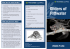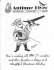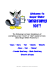# How to make dull cellular automata complex by Genaro J. Mart´ınez

## Transcription

How to make dull cellular automata complex by Genaro J. Mart´ınez
```How to make dull cellular automata complex by
adding memory: Rule 126 case study
Genaro J. Mart´ınez1,2 , Andrew Adamatzky2
Juan C. Seck-Tuoh-Mora3 , Ramon Alonso-Sanz2,4
December 16, 2009
1
2
Instituto de Ciencias Nucleares and Centro de Ciencias de la Complejidad,
Universidad Nacional Aut´onoma de M´exico, M´exico.
genaro[email protected]
Bristol Institute of Technology, University of the West of England, Bristol,
United Kingdom.
[email protected]
3
Centro de Investigaci´
Aut´
onoma del Estado de Hidalgo Pachuca, Hidalgo, M´exico.
[email protected]
4
ETSI Agr´
[email protected]
Abstract
Using Rule 126 elementary cellular automaton (ECA) we demonstrate
that a chaotic discrete system — when enriched with memory – hence exhibits complex dynamics where such space exploits on an ample universe
of periodic patterns induced from original information of the ahistorical
system. First we analyse classic ECA Rule 126 to identify basic characteristics with mean field theory, basins, and de Bruijn diagrams. In order
to derive this complex dynamics, we use a kind of memory on Rule 126;
from here interactions between gliders are studied for detecting stationary
patterns, glider guns and simulating specific simple computable functions
produced by glider collisions.
Keywords: elementary cellular automata, memory, Rule 126, gliders,
glider guns, filters, chaos and complex dynamics.
1
1
Introduction
In this paper we are making use of the memory tool to get a complex system
from a chaotic function in discrete dynamical environments. Such technique
takes the past history of the system for constructing its present and future: the
memory [9, 7, 3, 8, 4, 5]. It was previously reported in  how the chaotic ECA
Rule 30 is decomposed into a complex system applying memory on this function.
Recent results show that other chaotic functions (Rule 86 and Rule 101) yield
complex dynamics selecting a kind of memory, including a controller to obtain
self-organization by structure reactions and simple computations implemented
by soliton reactions .
We focus this work on cellular automata (CA) evolving in one dimension, in
particular taking the well-known ECA where each function evaluates a central
cell and its two nearest neighbors (from left and right) and, every cell takes a
value from a binary alphabet. Such ECA were introduced by Wolfram and have
been widely studied in several directions .
Among such ECA, there is a set of functions evolving in chaotic global
behaviour where a number of cells remain unordered and their transitions have
a large number of ancestors . In this sense, special attention is given on a
chaotic one: the ECA Rule 126 introduced by Wolfram in . Particularly in
,1 it is commented that Rule 126 generates a regular language with average
growing faster than any polynomial time. This property can be analysed as
well by de Bruijn diagrams with additional features; in particular another partial
analysis with de Bruijn and subset diagrams was done by McIntosh  showing
that string 010 in Rule 126 is the minimal Garden of Eden configuration (having
no ancestors).
Based on the idea developed in previous results for obtaining complex dynamics from chaotic functions selecting memory and working systematically, it
was suspected that a complex dynamics may emerge in Rule 126 given its relation to regular languages; making use of gliders coded by regular expressions,
as it was studied in Rule 110  and Rule 54 .
In this way Rule 126 provides a special case of how a chaotic behaviour
can be decomposed selecting a kind of memory into a extraordinary activity of
gliders, glider guns, still-life structures and a huge number of reactions. Such
features can be compared to Brain Brian’s rule behaviour or Conway’s Life but
in one dimension; actually none traditional ECA could have a glider dynamics
comparable to the one revealed in this ECA with memory denoted as φR126maj
(following notation described in [18, 17]).
1.1
One-dimensional cellular automata
One-dimensional CA is represented by an array of cells xi where i ∈ Z (integer
set) and each x takes a value from a finite alphabet Σ. Thus, a sequence of
cells {xi } of finite length n describes a string or global configuration c on Σ.
1 http://mathworld.wolfram.com/Rule126.html
2
This way, the set of finite configurations will be expressed as Σn . An evolution
is comprised by a sequence of configurations {ci } produced by the mapping
Φ : Σn → Σn ; thus the global relation is symbolized as:
Φ(ct ) → ct+1
(1)
where t represents time and every global state of c is defined by a sequence of
cell states. The global relation is determined over the cell states in configuration
ct updated at the next configuration ct+1 simultaneously by a local function ϕ
as follows:
ϕ(xti−r , . . . , xti , . . . , xti+r ) → xt+1
.
i
(2)
Wolfram represents one-dimensional CA with two parameters (k, r), where
k = |Σ| is the number of states, and r is the neighbourhood radius, hence ECA
domain is defined by parameters (2, 1). There are Σn different neighbourhoods
n
(where n = 2r + 1) and k k distinct evolution rules. The evolutions in this
paper have periodic boundary conditions.
1.2
Cellular automata with memory
Conventional CA are ahistoric (memoryless): i.e., the new state of a cell depends
on the neighbourhood configuration solely at the preceding time step of ϕ. CA
with memory can be considered as an extension of the standard framework of
CA where every cell xi is allowed to remember some period of its previous
evolution. Basically memory is based on the state and history of the system,
thus we design a memory function φ, as follows:
φ(xt−τ
, . . . , xt−1
, xti ) → si
i
i
(3)
such that τ < t determines the backwards degree of memory and each cell si ∈ Σ
is a function of the series of states in cell xi up to time-step t − τ . Finally to
execute the evolution we apply the original rule again as follows:
ϕ(. . . , sti−1 , sti , sti+1 , . . .) → xt+1
.
i
In CA with memory, while the mapping ϕ remains unaltered, a historic
memory of past iterations is retained by featuring each cell as a summary of its
previous states; therefore cells canalize memory to the map ϕ. As an example,
we can take the memory function φ as a majority memory:
φmaj → si
(4)
where in case of a tie given by Σ1 = Σ0 in φ, we shall take the last value xi . So
φmaj represents the classic majority function for three variables , as follows:
φmaj : (x1 ∧ x2 ) ∨ (x2 ∧ x3 ) ∨ (x3 ∧ x1 ) → x
3
on cells (xt−τ
, . . . , xt−1
, xti ) and defines a temporal ring before calculating the
i
i
next global configuration c. In case of a tie, it is allows to break it in favor of
zero if xτ −1 = 0, or to one whether xτ −1 = 1. The representation of a ECA
with memory (given previously in [18, 17]) is given as follows:
φCARm:τ
(5)
where CAR represents the decimal notation of a particular ECA and m the
kind of memory given with a specific value of τ . Thus the majority memory
(maj) working in ECA Rule 126 checking tree cells (τ = 3) of history is simply
denoted as φR126maj:3 . Figure 1 depicts in detail the memory working on ECA.
classic ECA (ahistoric)
ECA with memory
t−τ
..
.
..
.
t−1
t
φm:τ
t
temporal ring storing memory
ϕ
{si }
ϕ
t+1
t+1
Figure 1: Cellular automata with memory in cells.
Note that memory is as simple as any CA local function but sometimes the
global behaviour produced by the local rule is totally unpredictable.
Disclaimer
The memory mechanism considered here differs from other CA with memory
previously reported, often referred as higher order (in-time) CA.2 These ones,
in most cases, explicitly alter the function φ and incorporate memory by direcly
determining the new configuration in terms of the configurations at previous
time-steps. Thus, in second order in time (memory of capacity two) rules, the
transition rule operates as: xt+1 = Φ(ct , ct−1 ). Double memory (in transition
rule and in cells) can be implemented as: xt+1 = Φ({st }, {st−1 }). Particularly
interesting is the reversible formulation: xt+1 = ϕ xt−1 ; reversible CA with
memory are studied in .
2 See,
for example,  p. 118;  p. 43; or class MEMO in  p. 7.
4
Some authors define rules with memory as those with dependence in ϕ on
the state of the cell to be updated [33, 14]. So one-dimensional rules with no
memory adopt the form: xt+1 = ϕ(xti−1 , xti+1 ). Memory is not here indentified
with delay, i.e., refering cells exclusively to their state values a number of timesteps in the past, . So, for example, the cell to be updated may be referenced
not at t but at t − 1: xt+1 = ϕ(xti−1 , xti , xti+1 ) . Again, the mapping function
is not extended, for example, to consider the influence of cell i at time t − 1:
xt+1 = ψ(xt−1
, xti−1 , xti , xti+1 ) as done in .
i
The use of the locution associative memory usually refers, when used in the
CA context, to the study of configuration attractors [11, 19], which are argued
by Wuensche  to constitute the network’s global states contents addressable
memory in the sense of Hopfield .
Finally, it is not intended here to emulate human memory, i.e., the associative, pattern matching, highly parallel function of human memory. The aim is
just to store the past, or just a part of it, to make it work in dynamics. Thus,
working storage might replace here the use of the term memory, avoiding the
anthropomorphic, and rather unavoidable, connotations of the word memory
.
2
The basic function: ECA Rule 126
The local-state transition function ϕ corresponding to Rule 126 is represented
as follows:
1 if 110, 101, 100, 011, 010, 001
ϕR126 =
.
0 if 111, 000
(a)
(b)
Figure 2: Typical fractal (a) and chaotic (b) global evolution of ECA Rule 126.
(a) initially all cells in ‘0’ but one in state ‘1,’ (b) evolution from random initial
configuration with 50% of ‘0‘ and ‘1’ states.
Rule 126 has a chaotic global behaviour typical from Class III in Wolfram’s
classification . In ϕR126 we can easily recognize an initial high probability
5
of alive cells, i.e. cells in state ‘1’; with a 75% to appear in the next time and,
complement of only 25% to get state 0. It will be always a new alive cell iff
ϕR126 has one or two alive cells such that the equilibrium comes when there is
an overpopulation condition. Figure 2 shows these cases in typical evolutions
of Rule 126, both evolving from a single cell in state ‘1’ (fig. 2a) and from a
random initial configuration (fig. 2b) where a high density of 1’s is evidently in
the evolution.
While looking on chaotic space-time configuration in fig. 2 we understand the
difficulty for analysing the rule’s behaviour and selecting any coherent activity
among periodic structures without special tools.
2.1
Mean field approximation in ECA Rule 126
This section presents a probabilistic analysis with mean field theory, in order
to search basic properties about ϕR126 evolution space and its related chaotic
behaviour. Such analysis will offer a better spectrum where we can start to
explore the evolution space from more useful and specific initial conditions where
some interesting behaviours may emerge.
Mean field theory is a well-known technique for discovering statistical properties of CA without analysing evolution spaces of individual rules . The
method assumes that states in Σ are independent and do not correlate each
other in the local function ϕR126 . Thus we can study probabilities of states in a
neighbourhood in terms of the probability of a single state (the state in which
the neighbourhood evolves), and probability of the neighbourhood is product of
the probabilities of each cell in it.
In this way,  presents an explanation of Wolfram’s classes by a mixture
of probability theory and de Bruijn diagrams, resulting a classification based on
mean field theory curve:
• class I: monotonic, entirely on one side of diagonal;
• class II: horizontal tangency, never reaches diagonal;
• class IV: horizontal plus diagonal tangency, no crossing;
• class III: no tangencies, curve crosses diagonal.
For the one-dimensional case, all neighbourhoods are considered as follows:
pt+1 =
k2r+1
X−1
ϕj (X)pvt (1 − pt )n−v
(6)
j=0
such that j is an index number relating each neighbourhood and X are cells
xi−r , . . . , xi , . . . , xi+r . Thus n is the number of cells into every neighbourhood,
v indicates how often state ‘1’ occurs in X, n−v shows how often state ‘0’ occurs
in the neighbourhood X, pt is the probability of cell being in state ‘1’ while qt
is the probability of cell being in state ‘0’ i.e., q = 1 − p. The polynomial for
Rule 126 is defined as follows:
6
pt+1 = 3pt qt .
(7)
Because ϕR126 is classified as a chaotic rule, we expect no tangencies and its
curve must cross the identity; remembering that ϕR126 has a 75% of probability
to produce a state one.
Figure 3: Mean field curve for ECA Rule 126.
Mean field curve (fig. 3) confirms that probability of state ‘1’ in space-time
configurations of ϕR126 is 0.75 for high densities related to big populations of 1’s.
The curve demonstrates also that ϕR126 is chaotic because the curve cross the
identity with a first fixed point at the origin f = 0 and the non existence of any
unstable fixed point inducing non stable regions in the evolution. Nevertheless,
the stable fixed point is f = 0.6683, which represents a ‘concentration’ of ‘1’s
diminishing during the automaton evolution.
So the initial inspection indicates no evidence of complex behaviour emerging
in ϕR126 . Of course a deeper analysis is necessary for obtaining more features
from a chaotic rule, so the next sections explain other techniques to study in
particular periodic structures.
2.2
Basins of attraction
A basin (of attraction) field of a finite CA is the set of basins of attraction into
which all possible states and trajectories will be organized by the local function
ϕ. The topology of a single basin of attraction may be represented by a diagram,
the state transition graph. Thus the set of graphs composing the field specifies
the global behaviour of the system .
Generally a basin can also recognize CA with chaotic or complex behaviour
following previous results on attractors . Thus we have that Wolfram’s
classes can be represented as a basin classification:
7
• class I: very short transients, mainly point attractors (but possibly also periodic attractors) very high in-degree, very high leaf density (very ordered
dynamics);
• class II: very short transients, mainly short periodic attractors (but also
point attractors), high in-degree, very high leaf density;
• class IV: moderate transients, moderate-length periodic attractors, moderate in-degree, very moderate leaf density (possibly complex dynamics);
• class III: very long transients, very long periodic attractors, low in-degree,
low leaf density (chaotic dynamics).
(a)
(b)
(c)
(d)
Figure 4: Periodic patterns from some basin attractors.
The basins depicted in fig. 5 show the whole set of non-equivalent basins in
Rule 126 from l = 2 to l = 18 (l means length of array) attractors, all they
display not high densities from an attractor of mass one and attractors of mass
14.3 This way Rule 126 displays some non symmetric basins and some of them
have long transients that induce a relation with chaotic rules.
Particularly we can see specific cycles in fig. 4 where it is possible to find:
(a) static configurations as still life patterns (l = 8);
(b) traveling configurations as gliders (l = 15);
(c) meshes (l = 12);
(d) or empty universes (l = 14).
The cycle diagrams expose only displacements to the left, and this empty
universe evolving to the stable state 0 is constructed all times on the first basin
for each cycle, see fig. 5.
This way some cycles could induce some non trivial activity in Rule 126,
but the associated initial conditions are not generally predominant. However
3 Basins and attractors were calculated with Discrete Dynamical System DDLab available
from http://www.ddlab.org/
8
18.
17.
16.
15.
14.
13.
12.
11.
10.
9.
2.
3.
4.
5.
6.
7.
8.
Figure 5: The whole set of non-equivalent basins in ECA Rule 126 from l = 2
to l = 18.
9
some information is useful indeed looking periodic patterns that have a high
frequency inside this evolution space and hence for recognizing a kind of filter
useful to get a better view of a possible complex activity in Rule 126.
2.3
De Bruijn diagrams
De Bruijn diagrams [23, 30] are very adequate for describing evolution rules in
one-dimensional CA, although originally they were used in shift-register theory
(the treatment of sequences where their elements overlap each other). Paths in
a de Bruijn diagram may represent chains, configurations or classes of configurations in the evolution space.
For an one-dimensional CA of order (k, r), the de Bruijn diagram is defined
as a directed graph with k 2r vertices and k 2r+1 edges. The vertices are labeled
with the elements of the alphabet of length 2r. An edge is directed from vertex
i to vertex j, if and only if, the 2r − 1 final symbols of i are the same that the
2r − 1 initial ones in j forming a neighbourhood of 2r + 1 states represented by
i j. In this case, the edge connecting i to j is labeled with ϕ(i j) (the value
of the neighbourhood defined by the local function) .
The de Bruijn diagram associated to Rule 126 is depicted in fig. 6.4
0
0
0
3
1
1
1
1
1
1
2
1
Figure 6: De Bruijn diagram for the ECA Rule 126.
Figure 6 exposes that there are two neighbourhoods evolving into 0 and six
neighbourhoods into 1; so the higher frequency is for state 1; indicating the
possibility of having an injective automaton; that is, the existence of Garden of
Eden configurations [23, 30]. Classical analysis in graph theory has been applied
over de Bruijn diagrams for studying topics such as reversibility ; in other
sense, cycles in the diagram indicate periodic constructions in the evolution
of the automaton if the label of the cycle agrees with the sequence defined
by its nodes, taking periodic boundary conditions. Let us take the equivalent
construction of a de Bruijn diagram in order to describe the evolution in two
steps of Rule 126 (having now nodes composed by sequences of four symbols);
the cycles of this new diagram are presented in fig. 7.
4 De Bruijn and subset diagrams were calculated using NXLCAU21 designed by Harold V.
McIntosh; available from http://delta.cs.cinvestav.mx/~mcintosh
10
The extended de Bruijn diagrams  are useful for calculating all periodic
sequences by the cycles defined in the diagram. These ones also show the shift
of a sequence for a certain number of generations. Thus we can get de Bruijn
diagrams describing periodic sequences for Rule 126.
(a)
(b)
(c)
Figure 7: Patterns calculated from extended de Bruijn diagrams, in particular
from cycles of order (x, 2) (that means a x-shift in y-generations).
Cycles inside de Bruijn diagrams can be used for obtaining regular expressions representing a periodic pattern. Figure 7 displays three patterns calculated
as: (a) shift −3 in 2 generations representing a pattern with displacement to
the left, (b) shift 0 in 2 generations describing a static pattern traveling without
displacement, and (c) shift +3 in 2 generations is exactly the symmetric pattern
given in the first evolution.
So we can also see in fig. 7 that it is possible to find patterns traveling in both
directions, as gliders or mobile structures. But generally these constructions
(strings) cannot live in combination with others structures and therefore it is
really hard to have this kind of objects with such characteristics. Although,
moreover Rule 126 has at least one glider! This will be explained in the next
sections.
2.4
Filters for recognizing dynamics in Rule 126
Filters are a useful tool for discovering hidden order in chaotic or complex
rules. Filters were introduced in CA studies by Wuensche who employed them
to automatically classify cell-state transition functions, see . Also filters
related to tiles were successfuly applied and deduced in analysing space-time
behaviour of ECA governed by Rules 110 and 54 [25, 20, 21].
This way, we have found that Rule 126 has two types of two-dimensional
tiles (which together work as filters over ϕR126 ):
»
–
• the tile t1 = 1111
, and
1001
11
3
0000000
601111107
6
7
t2 = 611000117.
401101105
1111111
2
• the tile
Filter t1 works more significantly on configurations generated by ϕR126 , the
second one is not frequently found although it is exploited when Rule 126 is
altered with memory (as we can see in following sections).
The application of the first filter is effective to discover gaps with little
patterns traveling on triangles of ‘1’ states in the evolution space. Although
even in this case it may be unclear how a dynamics would be interpreted, a
careful inspection on the evolution brings to light very small gliders (as still
life), as shown in fig. 8.
Figure 8: Filtered space-time configuration in ϕR126 .
This glider emerging in Rule 126 and localized by a filter is precisely the
periodic pattern calculated with the basin (fig. 4a) and the de Bruijn diagram
(fig. 7b); in particular the last one offers more information because such cycles
allow to classify the whole phases when this glider is coded in the initial condition. The next sections demonstrate the effect of filters for recognizing an
amazing universe evolving in this CA with memory.
3
CA φR126m:4 and complex dynamics
This section discusses both relevant aspects of classic Rule 126 (ϕR126 ) and Rule
126 with memory (φR126m:τ ).
3.1
Dynamics emerging with majority memory
As it was explained in [6, 18] a new family of evolution rules derived from classic
ECA can be found selecting a kind of memory.
12
Figure 9 illustrates dynamics for some values of τ in φR126maj .5 The spacetime configurations are also filtered to show a raw dynamics. We found that
large odd values of τ tend to define macrocells-like patterns [36, 23]. Even values
of τ are responsible of a mixture of periodic and chaotic dynamics.
original
τ =3
τ =4
τ =5
τ =6
τ =7
τ =8
τ =9
τ = 10
τ = 11
τ = 12
τ = 13
Figure 9: CA with majority memory φR126maj:τ where 13 values of τ are evolved
and filtered over a ring of 246 cells for 236 generations.
On exploring systematically distinct values of τ , we found that φR126maj:4
produces an impressive and non-trivial emergence of patterns traveling and colliding; so yet when the memory is working as a new function, it is possible to
recognize some fragments inherited of the original rule ϕR126 .
We start our simulations with a single non-quiescent cell, an example of
this space-time configuration is provided in fig. 10 showing the first 1,156 steps,
where in this case the automaton needed other 12,000 steps to reach a stationary
configuration. Filter is convenient to eliminate the non relevant information
about gliders. In fig. 10 we can see a number of gliders, glider guns, still-life
configurations, and a wide number of combinations of such patterns colliding and
traveling with different velocities and densities. Consequently we can classify a
number of periodic structures, objects and interesting reactions.
5 Evolutions of φ
R126maj:τ were calculated with OSXLCAU21 system available in http:
//uncomp.uwe.ac.uk/genaro/OSXCASystems.html
13
Figure 10: Filtered space-time configuration of φR126maj:4 with 602 cells, periodic boundaries, starting from one non-quiescent cell and running for 1,156
steps.
14
Basic primitive gliders are displayed in fig. 11, there are still-life patterns s1
and s2 , and gliders g1 and g2 respectively. These structures can be ordered in
a set G φR126maj:4 = {s1 , s2 , g1 , g2 }.
s1
g1
s2
g2
Figure 11: Basic gliders in φR126maj:4 . Two stationary configurations (as still
life patterns) s1 and s2 respectively, and two gliders g1 and g2 .
structure
s1
s2
g1
g2
gun1
gun2
gun3
gun4
vg
0/2 = 0
0/10 = 0
3/5 ≈ 0.6
−3/5 ≈ −0.6
0/19 = 0
0/27 = 0
0/110 = 0
0/84 = 0
lineal volume
1
12
8
8
6
6
10
15
mass
1
28
17
17
-
Table 1: Properties of gliders G φR126maj:4 . Velocity vg is calculated as displacement between period. The linear volume is the maximum distance between two
cells in state ‘1’ (diameter of the set of cells in state ‘1’), finally mass is the
number of cells in state ‘1.’
Figure 12 shows the three more frequent glider guns (gun1 , gun2 , and gun3 )
emerging in φR126maj:4 . The next three guns (fig. 12(a), (b) and (c)) are combined in basic guns synchronized by multiple reactions which preserve emission
of gliders although some of them can get other frequencies. We can include them
increasing the set of periodic structures G φR126maj:4 = {s1 , s2 , g1 , g2 , gun1 , gun2 ,
gun3 , gun4 }. The last gun is presented in fig. 14 as a collision of gliders.
Basic properties of G φR126maj:4 are given in table 1, where also glider guns
can be classified by frequencies of glider emission as follows:
• small gun1 fires a gliders g1 and g2 (in turn) every five steps;
• medium gun2 fires g1 and g2 (in turn) every 26 steps;
• large gun3 fires five g1 and g2 gliders every 100 steps.
Frequencies are related to the number of emitted gliders (in intervals) by a
glider gun. Hence the gun2 generates one g1 and another g2 glider eight steps
15
gun1
gun2
gun3
(a)
(b)
(c)
Figure 12: Samples of glider guns and their compositions in φR126maj:4 .
before to produce the next ones, and a gun3 yields five g1 and five g2 gliders
100 steps before to produce the other ones.
3.2
Collisions between gliders
Coding glider positions to get a desired reaction is a well-known solution for
some related problems about complex behaviours. One of them is precisely the
problem of self-organization (by structures fig. 13) . In this way, we present
how each basic glider can be produced by collisions between other different
gliders.
(a)
(b)
(c)
(d)
(e)
(f)
Figure 13: Generating basic gliders from collisions between other ones, this
is self-organization by structures. The following reactions are illustrated as
follows: (a) g1 + g2 = s1 , (b) s1 + g2 = g1 , (c) g1 + s1 = g2 , (d) s2 + g2 = g1 , (e)
g2 + s1 = g2 , and (f) g1 + g2 = s2 .
A little bit more complicated is to obtain glider guns by collisions. Figure 14(a) and (b) depicts the production of a gun2 from a multiple collision of
16
(a)
(b)
(c)
(d)
(e)
Figure 14: Generating gliders guns by collisions.
gliders. Later on we shall present two new combinations of guns (fig. 14(c) and
(e)) and a new gun4 (fig. 14(d)).
3.3
Other collisions
(a)
(b)
(c)
(d)
(e)
Figure 15: Eater reactions between gliders and still lives (a, b, and c) and
annihilation of gliders by collisions (d and e).
A large variety of objects can be generated by collisions between gliders in
φR126maj:4 . Some of these objects are useful for designing complex dynamical
structures. For instance, fig. 15(a), (b) and (c) shows how to delete (as an eater
17
configuration) a single glider or a stream of gliders g1 and g2 . Two kinds of
annihilations are depicted as well (see fig. 15(d) and (e)).
Figure 16: Black hole patterns consuming g1 and g2 gliders.
Figure 17: Examples of soliton reactions.
Figure 16 describes a number of black hole patterns emerging in φR126maj:4 .
Traditionally a black hole is a Life object6 absorbing any glider that comes
close to the main body (in this case, still life patterns). Its relevance consists of
knowing how many patterns are able of attracting gliders and consuming all of
them forever, in these cases the g1 and g2 gliders.
(a)
(b)
(c)
(d)
(e)
(f)
Figure 18: Examples of other collisions.
Figure 17 displays soliton reactions, where colliding gliders preserve their
original forms. As known, a soliton has a small change in their phase and
displacement; also soliton reactions are obtained with single gliders or by a
stream of them.
6 You can see a large classification of Life objects in http://www.conwaylife.com/wiki/
index.php
18
Figure 19: Table of binary, multiple and other collisions.
19
binary
s1 ← g2
g1 → s1
s1 ← g2
g1 → s1
s2 ← g2
g1 → s2
g1 ↔ g2
g1 ↔ g2
g1 ↔ g2
g1 ↔ g2
g1 ↔ g2
g1 ↔ g2
g1 ↔ g2
g1 ↔ g2
g1 ↔ g2
= s1
= s1
= g1
= g2
= g1
= g2
=∅
= s1
= s2
= g1
= g2
= 2g1
= 2g2
= g2 + 2g1
= g1 + 2g2
Summary of collisions
multiple
s2 ← g2 = 2g1
g1 → s2 = 2g2
s2 ← 2g2 = 2g1
2g1 → s2 = 2g2
s2 ← 2g2 = 2g1 + g2
2g1 → s2 = g1 + 2g2
2g1 ↔ 2g2 = ∅
2g1 ↔ 2g2 = g1
2g1 ↔ 2g2 = g2
2g1 ↔ 2g2 = 2g1
2g1 ↔ 2g2 = 2g2
g1 ↔ 2g2 = g1
2g1 ↔ g2 = g2
g1 ↔ 2g2 = g2
2g1 ↔ g2 = g1
g1 ↔ 2g2 = ∅
2g1 ↔ g2 = ∅
g1 ↔ 2g2 = 2g2 + g1
2g1 ↔ g2 = g2 + 2g1
g1 ↔ 2g2 = 2g2 + 2g1
g1 ↔ 2g2 = 2g2 + g2 + 2g1
2g1 ↔ g2 = 2g2 + 2g1
2g1 ↔ g2 = 2g2 + 2g1 + g1
3g1 ↔ 3g2 = 2g1
g1 → s1 ← g2 = ∅
g1 → s1 ← g2 = s1
g1 → 2s2 ← g2 = g1 + g2
g1 → 2s2 ← g2 = g2 + g1
2g1 → s1 ← 2g2 = 2g2 + 2s2 + 2g1
3g1 ↔ 3g2 = g2 + 2g2 + 2g1
4g1 ↔ g2 = 2g1 + g1
g1 ↔ 4g2 = g2 + 2g2
in φR126maj:4
soliton
g1 → s1 = s1 + g1
s1 ← g2 = g2 + s1
2g1 → s2 = s2 + 2g1
s2 ← 2g2 = 2g2 + s2
2g1 → 2s2 = 2g1 + 2s2
2s2 ← 2g2 = 2g2 + 2s2
2g1 ↔ 2g2 = 2g2 + 2g1
(∗ means gun composed)
guns
s1 ← 2g2 = gun1
gun2 ← g2 = gun1
g1 ↔ g2 ← 2g2 = gun2
g1 ↔ g2 = gun3
2g1 ↔ 2g2 = gun2 ∗
3g1 ↔ 3g2 = gun1 ∗
The last set of examples (fig. 18) presents other binary reactions and some
multiple collisions between g1 and g2 gliders. Some of them are conservative
and others produce a new number of these structures. Finally we can take
some collisions to exploit their dynamics and controlling glider reactions (see
an extended relations of collisions in table 19). Thus it can be obtained a full
number of reactions for generating desired gliders. The set of collisions is useful
as ‘raw material’ in the implementation of computations on φR126maj:4 .
4
Computing in φR126maj:4
Given the large number of reactions in φR126maj:4 (see table 19) the rule could
be useful for implementing collision-based computing schemes [2, 20].
a
¬a ∧ b
b
a∧b
a ∧ ¬b
Figure 20: Colliding interactions deriving in logic gates.
Figure 20 illustrates the interaction of gliders traveling, colliding one another
and implementing a Boolean conjunction as result. Initially from previous collisions we can embed logical constructions of and and not gates from this figure,
as follows:
• for the gate (¬a ∧ b) the implementation with φR126maj:4 is in fig. 18(b);
but only with one g2 glider (see tab. 19).
• for the gate (a ∧ b) the implementation corresponds to fig. 13(a).
• for the gate (a ∧ ¬b) the implementation is presented in fig. 18(a); but
only with one g1 glider (see table 19).
• for one fanout gate (a ↔ b = a + a + b) the implementation is shown in
fig. 18(d).
Indeed, here we also adopt ideas developed by Rennard in his design of
Life computing architectures . Glider g1 represents value 0, two g1 gliders
together represent a value 1. Two gliders 2g2 traveling in positive direction
describe the operator and one the register. Thus the register will read false or
true if they are produced successfully.
Figure 21 illustrates the basic reactions required to produce a primitive computational scheme in φR126maj:4 . The following set of relations is applied (see
table 19):
20
2g2
g1
2g1
2g2
2g1
2g2
2g1
g1
2g1
g1
2g1
TRUE
FALSE
REGISTER
Figure 21: Example of devices working as data, operator and register respectively by gliders in G φR126maj:4 .
2g1 ↔ 2g2 = g1 ↔ 2g2 = g1
2g1 ↔ 2g2 = g1
g1 ↔ 2g2 = 2g2 + g1
2g1 ↔ 2g2 = 2g2 + 2g1
so we can represent serial reactions as:
2g1 + 2g2 = 2g1 + 2g2 = g1
2g1 + 2g2 = 2g1
empty word
false
true.
and a not gate can be represented as:
• false+2g2 = true+2g2 , or
• true+2g2 = false+2g2 .
4.1
Constructing formal languages since gliders collisions
in φR126maj:4
To be considered as a mathematical machine, φR126maj:4 should compute sets
of formal languages . We consider such implementation as an easy way to
illustrate how implement some collision-based processes in φR126maj:4 .
Let Σ be a non-empty finite alphabet. Thus a string over Σ is a finite
sequence of symbols from Σ. A set of all strings over Σ of length n is denoted by Σn . For example, if Σ = {0, 1}, then Σ2 = {00, 01, 10, 11} to Σ3 =
{000, 001, 010, 011, 100, 101, 110, 111}, and so on. This way Σ0 = {} for any
alphabet Σ. The set of all strings over Σ of any length is the Kleene closure of
Σ and is denoted as Σ∗ . However we can write such expression in terms of Σn ,
as follows:
[
Σ∗ =
Σn .
(8)
n∈Z0
This way for a binary alphabet Σ = {, 0, 1, 00, 01, 10, 11, 000, 001, . . .}. Finally a set of strings on Σ is called a formal language.
∗
21
!
0
1
0
0
0
1
1
1
0
1
Figure 22: Constructing the formal collision-based languages in φR126maj:4 :
They are Σ0 (top), Σ1 (middle), and Σ2 (bottom).
Making use of gliders in φR126maj:4 we can get any set of strings of Σ∗ . For
example, for the formal language 1∗ we need to code all the initial conditions
with reactions true. For the formal language (00 + 11)∗ the initial condition
will be coded as: (g1 g1 + 2g1 2g1 )∗ . To yield an arbitrary length of strings we
increase the number of cells in the CA. All distances between gliders must be
preserved and the left part become periodic.
5
Conclusions
We have enriched ECA Rule 126 with majority memory and have demonstrated
that by applying certain filtering procedures we can extract rich dynamics of
gliders, guns and infer a sophisticated system of reactions between gliders. We
have discovered how a complex dynamics emerges from a chaotic system selecting an adequate memory. We have shown that the majority memory increases
nominal complexity but decreases statistical complexity of patterns generated
by the CA. By applying methods as de Brujin diagrams, cycles and graph theory,
22
we have proved that Rule 126 with memory opens a new spectrum of complex
rules, in this case a new CA with memory: φR126maj:4 . Finally we have demonstrated some capacities for computing specific logical and memory functions,
and a future work will be constructing a universal device.
Acknowledgement
Genaro J. Mart´ınez and Ramon Alonso-Sanz are supported by EPSRC (grants
EP/F054343/1 and EP/E049281/1). Juan C. Seck-Tuoh-Mora is supported by
CONACYT (project CB-2007/83554).
References
 Adamatzky, A. (1994) Identification of Cellular Automata, Taylor and
Francis.
 Adamatzky, A. (Ed.) (2003) Collision-Based Computing, Springer.
 Alonso-Sanz, R. (2003) Reversible Cellular Automata with Memory, Physica D 175 1–30.
 Alonso-Sanz, R. (2006) Elementary rules with elementary memory rules:
the case of linear rules, Journal of Cellular Automata 1 71–87.
 Alonso-Sanz, R. (2009) Memory boosts cooperation in the structurally dynamic prisoner’s dilemma, Int. J. Bifurcation and Chaos, in press.
 Alonso-Sanz, R. (2009) Cellular Automata with Memory, Old City Publishing.
 Alonso-Sanz, R. & Martin, M. (2002) One-dimensional cellular automata
with memory: patterns starting with a single site seed, Int. J. Bifurcation
and Chaos 12 205–226.
 Alonso-Sanz, R. & Martin, M. (2003) Elementary CA with memory, Complex Systems 14 99–126.
 Alonso-Sanz, R., Martin, M.C., & Martin, M. (2001) The effect of memory
in the spatial continuous-valued prisoner’s dilemma, Int. J. Bifurcation and
Chaos 11 (8) 2061–2083.
 Arbib, M.A. (1969) Theories of Abstract Automata, Prentice-Hall Series in
Automatic Computation.
 Ganguly, N., Maji, P., Das, A., Sikdar, B.P., & Chaudhury, P. (2002)
Generalized multiple attractor cellular automata (GMACA) model for associative memory, Int. J. of Bifurcation and Chaos 16 (7) 781–795.
23
 Hopfield, J.J. (1982) Neural networks amd physical systems with emergent
collective computational abilities, Proc. Nat. Acad. Sci. USA 79 2554–2558.
 Ilachinski, A. (2001) Cellular Automata. A Discrete Universe, World Scientific.
 Kauffman, S.A. (1984) Emergent propierties in random complex automata,
Physica D 10 145–156.
 Kauffman, S.A. (1993) The Origins of Order: Self-Organization and Selection in Evolution, Oxford University Press, New York.
 Letourneau,
P-J. (2007) http://demonstrations.wolfram.com/
OuterTotalistic2DCellularAutomataWithMemory/,
and
http:
//www.wolframscience.com/conference/2006/presentations/
materials/letourneau.pdf
 Mart´ınez, G.J., Adamatzky, A., & Alonso-Sanz, A., Complex dynamics of
cellular automata emerging in chaotic rules, Journal of Nonlinear Systems
and Applications, in press.
 Mart´ınez, G.J., Adamatzky, A., Alonso-Sanz, A., & Seck-Tuoh-Mora, J.C.,
Complex dynamics emerging in Rule 30 with majority memory, Complex
Systems 18 (3), in press.
 Maji, P. & Chaudhury, P. (2008) Non-uniform cellular automata based
associative memory: Evolutionary design and basins of attraction, Information Sciences 178 2315–2336.
 Mart´ınez, G.J., Adamatzky, A., & McIntosh, H.V. (2006) Phenomenology
of glider collisions in cellular automaton Rule 54 and associated logical
gates, Chaos, Solitons and Fractals 28 100–111.
 Mart´ınez, G.J., Adamatzky, A., & McIntosh, H.V. (2008) On the representation of gliders in Rule 54 by de Bruijn and cycle diagrams, Lecture Notes
in Computer Science 5191 83–91.
 McIntosh, H.V. (1990) Wolfram’s Class IV and a Good Life, Physica D 45
105–121.
 McIntosh, H.V. (2009) One Dimensional Cellular Automata, Luniver Press.
 Minsky, M. (1967) Computation: Finite and Infinite Machines, Prentice
Hall.
 Mart´ınez, G.J., McIntosh, H.V., & Seck Tuoh Mora, J.C. (2006) Gliders in
Rule 110, Int. J. of Unconventional Computing 2 (1) 1–49.
 Mart´ınez, G.J., McIntosh, H.V., Seck Tuoh Mora, J.C., & Chapa Vergara, S.V. (2008) Determining a regular language by glider-based structures called phases fi 1 in Rule 110, Journal of Cellular Automata 3 (3)
231–270.
24
 Rennard, J.P. (2003) Implementation of Logical Functions in the Game of
Life, 491–512 (in ).
 Rohlf, T. & Jost, C. (2009) A new class of cellular automata: How spatiotemporal delays affect dynamics and improve computation, In European
Conference on Complex Systems 2009.
 Seck-Tuoh-Mora, J.C., Chapa-Vergara, S.V., Mart´ınez, G.J., & McIntosh,
H.V. (2005) Procedures for calculating reversible one-dimensional cellular
automata, Physica D 202 134–141.
 Voorhees, B.H. (1996) Computational analysis of one-dimensional cellular
automata, World Scientific Series on Nonlinear Science, Series A, Vol. 15.
 Voorhees, B.H. (2008) Remarks on Applications of De Bruijn Diagrams
and Their Fragments, Journal of Cellular Automata 3 (3) 187–204.
 Wuensche, A. & Lesser, M. (1992) The Global Dynamics of Cellular Automata, Santa Fe Institute Studies in the Sciences of Complexity, AddisonWesley Publishing Company.
 Wolf-Gladrow, D.A. (2000) Lattice-gas Gellular Automata and Lattice
Boltzmann Models, Springer.
 Wolfram, S. (1983) Statistical Mechanics of Cellular Automata, Review
Modern Physics 55 601–644.
 Wolfram, S. (1984) Universality and complexity in cellular automata, Physica D 10 1–35.
 Wolfram, S. (1994) Cellular Automata and Complexity, Addison-Wesley
Publishing Company.
 Wolfram, S. (2002) A New Kind of Science, Wolfram Media, Inc., Champaign, Illinois.
 Wuensche, A. (1994) Complexity in one-d cellular automata, Santa Fe Institute working paper 94-04-025.
 Wuensche, A. (1994) The ghost in the machine: basins of attraction of
random Boolean networks, In Artificial Life III, Langton, C. (ed.), 465–
501.
 Wuensche, A. (1999) Classifying Cellular Automata Automatically, Complexity 4 (3) 47–66.
 Xuelog, Z., Qianmu, L., Manwu, X., & Fengyu, L. (2005) A symmetric
cryptography based on extended cellular automata, IEEE Int. Conf. on
Systems, Mand and Cybernetics 1 10–12.
25
```

### Japan State Dept #2009-131 - University of California, San Diego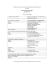### Newport Glider Health Care Series1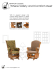### Universality and Complexity in Cellular Automata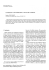### DPF or UREA### Sugar Glider Care and Husbandry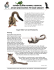### A Sugar Glider`s Cage Should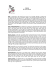### AMERICAN IS-PLACE WACO CG-4A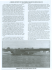### Gliders of Pittwater